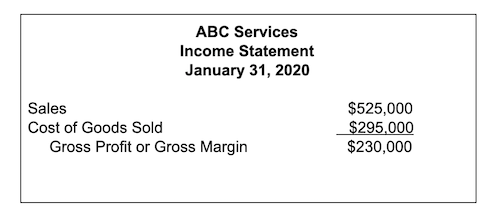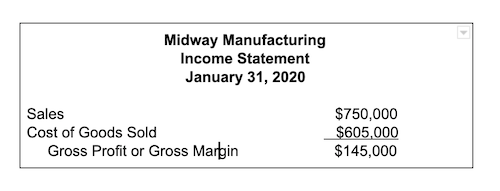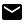# How to Calculate Gross Margin Ratio

Gross margin ratio is an important metric for your business. Easily calculated, gross margin ratio provides you with a quick snapshot of profitability.

Updated July 17, 2020

You’ve studied up on the most important accounting terms. You breezed through Accounting 101. Now it’s time to start analyzing profit levels for your business.

One of the best ways to look at sales profitability as well as the overall financial health of your business is by calculating gross margin ratio.

Gross margin looks at total revenue and total cost of goods sold to determine how much profit a company retains after direct costs are subtracted. Calculating your gross margin ratio provides the level of profitability of your business as a percentage.

## Overview: What is the gross margin ratio?

Though it may sound intimidating, calculating an important metric such as gross margin ratio or gross profit margin is really simple, with only a financial statement such as an income statement needed to complete the calculation process.

The gross margin ratio measures the amount of funds retained after products and services have been sold. The gross margin ratio is important because it focuses strictly on product/service profitability, essentially letting you know if you’re making enough money from selling your products and services.

### Gross margin ratio vs. net margin: What is the difference?

Both gross profit margin (also known as gross profit) and net profit margin (also known as net income) are used to establish how profitable a company is.

The major difference between the two calculations is that gross profit margin (or the gross margin ratio) concentrates exclusively on profitability from sales alone.

In other words, after calculating it, your profit margin ratio lets you know how much of each dollar received in sales is left over after the cost of goods sold is factored in, either as cents on the dollar or as a percentage.

Net margin, on the other hand, provides a snapshot of the profitability of the entire company, including not only the cost of goods sold in the equation, but all operating expenses as well.

## How to calculate gross margin ratio

The only data you’ll need to calculate the gross margin ratio is your total revenue and total cost of goods sold for the month (or whatever time frame you’re calculating your ratio for). The formula for calculating gross margin ratio percentage ratio is simple:

(Total Revenue - Cost of Goods Sold) ÷ Total Revenue x 100 = Gross Margin Ratio

For example, Joe’s Auto Shop recognized revenues in the amount of \$120,000 in the month of January, while his cost of goods sold was \$61,000. Remember, we’re not including any general expenses in this calculation, only expenses directly related to producing the goods and services.

Here’s how Joe would calculate his gross margin ratio:

(\$120,000 - \$61,000) ÷ \$120,000 x 100 = 49.16%

The above result means that for every dollar Joe’s Auto Shop brings in, 49.16% of it is available to pay for operations.

You can also calculate gross margin ratio as a dollar amount:

(\$120,000 - \$61,000) ÷ \$120,000 = 0.4916

The above gross margin formula indicates that for every dollar in revenue, \$0.49 is available for operational costs.

## Examples of gross margin ratio

Here are two examples of calculating gross margin ratio for hypothetical businesses.

Example A:Here is a hypothetical example of an Income Statement for ABC Services

ABC Building had sales in the amount of \$525,000, while cost of goods sold totaled \$295,000 for the month. You already have your gross margin simply by subtracting the cost of goods sold from sales. In order to calculate your gross margin ratio, you would complete the following calculation:

(\$525,000 - \$295,000) ÷ \$525,000 x 100 = 43.81%

This means 43.81% of every dollar earned can go toward operating expenses.

To calculate the above information as a dollar amount, use this calculation:

(\$525,000 - \$295,000) ÷ \$525,000 = \$0.44

This means \$0.44 of every dollar in sales can go toward operating costs.

Example B:Here is a hypothetical example of an Income Statement for Midway Manufacturing

In this example, Midway Manufacturing has sales in the amount of \$750,000, while cost of goods sold was \$605,000, leaving the company a gross margin of \$145,000. To calculate gross margin ratio, we would need to calculate the above information as follows:

(\$750,000 - \$605,000) ÷ \$750,000 x 100 = 19.33%

This means 19.33% of every dollar earned is retained for operating expenses.

To calculate the above information as a dollar amount, use this calculation:

(\$750,000 - \$605,000) ÷ \$750,000 = \$0.19

For every dollar Midway Manufacturing earns, it retains \$0.19.

You can see that the gross margin ratio varies widely between these two companies: ABC Services has 43.81% of its earnings available for operations, while Midway Manufacturing only has \$19.33% available.

While it appears that Midway Manufacturing is in worse financial condition than ABC Services, you should only compare gross margin ratios within your industry.

ABC Services is a service company, and Midway Manufacturing is a manufacturing company, so the two should only be compared to other similar companies within their own particular niche, not each other.

## What can you learn from calculating gross margin ratio?

Taking a few moments to perform this simple calculation can indicate how efficient your current business operations are, if you’re performing within industry standards, and if you need to revisit processes or pricing levels in order to become more profitable.

Gross margin ratio also helps you determine the profitability of the goods and services your business provides. Finally, calculating your gross margin ratio on a regular basis can help you spot trends and alert you to any significant changes before they become major issues.

If you’re looking for an easier way for you or your bookkeeper to manage your business finances, be sure to check out The Blueprint’s accounting software reviews to read our assessment of some of the most popular accounting software applications on the market today.

### LOTS TO CONSIDER, LET US HELP

Get The Blueprint’s latest recommendations by signing up to our free newsletter.The Motley Fool has a Disclosure Policy. The Author and/or The Motley Fool may have an interest in companies mentioned.